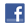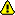New account
4 million accounts created!

• Home
• Report a bug

•

Learn German > German lessons and exercises > German test #67126> Other German exercises on the same topic: Numbers [Change theme] > Similar tests: - Numbers: 0-100 - Counting from 1 to 10 - Numbers from 20 to 100 - Numbers from 11 to 20 - Maths - Numbers - Counting from 100 to 1 000 - 100-100,000,000,000 > Double-click on words you don't understand

# Maths

Read this lesson :

 eins = onezwei = twodrei = threevier = fourfünf = fivesechs = sixsieben = sevenacht = eight neun = ninezehn = ten elf = elevenzwölf = twelvedreizehn = thirteenvierzehn = fourteenfünfzehn = fiveteensechzehn = sixteensiebzehn = seventeenachtzehn = eignteenneunzehn = nineteenzwanzig = twenty einundzwanzig = twenty onezweiundzwanzig = twenty two ..... Eins plus eins ist zwei = 1 + 1 = 2Vier minus zwei ist zwei = 4 - 2 = 2 Zwei mal zwei ist vier = 2 x 2 = 4Vier durch zwei ist zwei = 4: 2 = 2

Complete in lettersTwitter Share
German exercise "Maths" created by dada30400 with The test builder. [More lessons & exercises from dada30400]
Click here to see the current stats of this German testPlease log in to save your progress.

To insert special letters:

- Sieben plus zwei ist =

- Acht minus drei ist =

- Drei x ist neun.

- durch drei ist zwei.

- Eins plus ist acht.

- Vier durch vier ist .

- Acht minus vier ist .

- Zehn minus ist acht.

- Vier plus drei ist .

- Zwanzig durch zwei ist .

End of the free exercise to learn German: Maths
A free German exercise to learn German.
Other German exercises on the same topic : Numbers | All our lessons and exercises